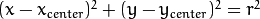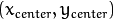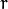#### Previous topic

Hough Line Transform

#### Next topic

Image Segmentation with Watershed Algorithm

# Hough Circle Transform¶

## Goal¶

In this chapter,
• We will learn to use Hough Transform to find circles in an image.
• We will see these functions: cv2.HoughCircles()

## Theory¶

A circle is represented mathematically aswhereis the center of the circle, andis the radius of the circle. From equation, we can see we have 3 parameters, so we need a 3D accumulator for hough transform, which would be highly ineffective. So OpenCV uses more trickier method, Hough Gradient Method which uses the gradient information of edges.

The function we use here is cv2.HoughCircles(). It has plenty of arguments which are well explained in the documentation. So we directly go to the code.

```import cv2
import numpy as np

img = cv2.medianBlur(img,5)
cimg = cv2.cvtColor(img,cv2.COLOR_GRAY2BGR)

circles = np.uint16(np.around(circles))
for i in circles[0,:]:
# draw the outer circle
cv2.circle(cimg,(i,i),i,(0,255,0),2)
# draw the center of the circle
cv2.circle(cimg,(i,i),2,(0,0,255),3)

cv2.imshow('detected circles',cimg)
cv2.waitKey(0)
cv2.destroyAllWindows()
```

Result is shown below: